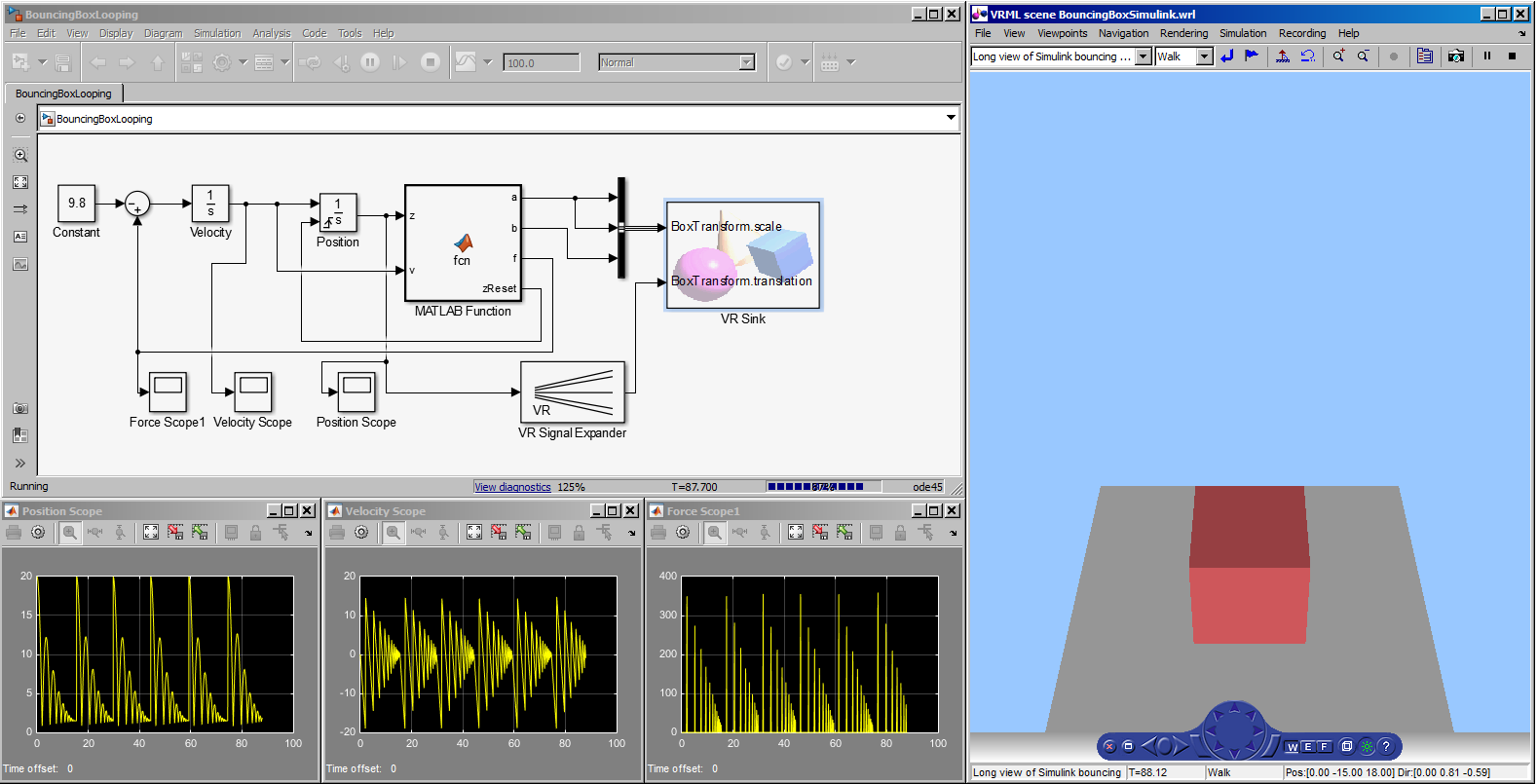Matlab and Simulink are a widely used mathematical modeling and simulation products.

See: Description

• Class Summary
Class DescriptionThe Matlab toolkit from Mathworks includes numerous mathematical and scientific packages. Simulink can be used for animation visualization using VRML models, which are easily created from X3D. X3D models can be converted to VRML for use with Simulink and other Matlab tools. Web3D 2015 conference resources: Simulink demo, poster paper, Fast Forward slides (.pdf) and video.